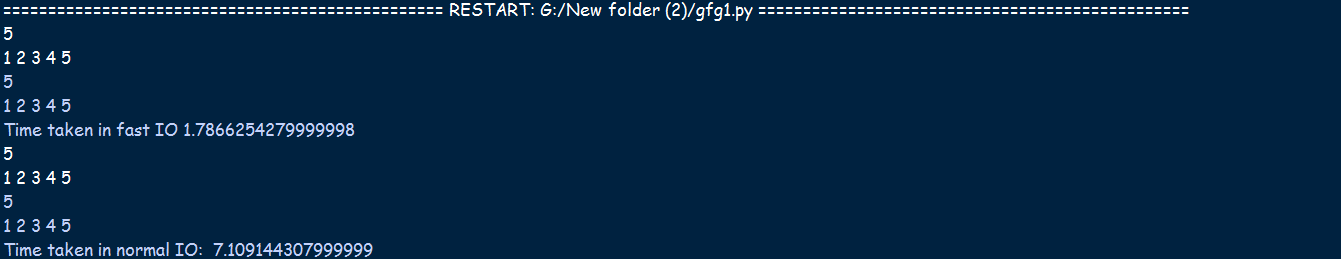# Avoid TLE in Python in Competitive Programming

• Difficulty Level : Easy
• Last Updated : 18 Jan, 2021

Reasons behind getting TLE:

• It is slower compared to other programming languages.
• It offers slower Input/Output.
• It has a lower recursion depth which often gives TLE in recursion and graph problems.

Tips to avoid TLE with Python:

### Convert to an Iterative Approach:

• Any problem of recursion can be converted to an iterative approach, so try to use the iterative approach instead of recursion.
• Using Stack and while loop may save the execution time of the program.

Program 1:

Given below is the code showing the time required for calculating the factorial of a number in both approaches:

## Python3

 `# Program to show the time taken in``# iteration and recursion`` ` `import` `time`` ` `# Recursive function to find factorial``# of the given number N``def` `factorial(N):``   ` `      ``# Base Case``    ``if` `N ``=``=` `0``:``        ``return` `1``       ` `    ``# Recursive Call``    ``return` `N ``*` `factorial(N ``-` `1``)`` ` `# Driver Code``if` `__name__ ``=``=` `'__main__'``:``    ``n ``=` `20`` ` `    ``# Find the time taken for the``    ``# recursive approach``    ``start ``=` `time.perf_counter()``    ``print``(``"Calculated using recursion: "``, factorial(n))``     ` `    ``end ``=` `time.perf_counter()``    ``print``(``"Time taken in recursion: "``, ``"{0:.9f}"``.``format``(end``-``start))`` ` `    ``# Find time taken for iterative``    ``# approach``    ``start ``=` `time.perf_counter()``    ``result ``=` `1``     ` `    ``while` `n > ``0``:``        ``result ``*``=` `n``        ``n ``-``=` `1``         ` `    ``print``(``"Calculated using the iterative method: "``, result)``     ` `    ``end ``=` `time.perf_counter()``    ``print``(``"Time taken in iteration: "``, ``"{0:.9f}"``.``format``(end``-``start))`

Output:

```Calculated using recursion:  2432902008176640000
Time taken in recursion:  0.000015925
Calculated using the iterative method:  2432902008176640000
Time taken in iteration:  0.000009279
```

### Set the recursion limit:

Syntax: sys.setrecursionlimit(limit)

Parameter: limit: An integer value that can be used to set the limit of the Python interpreter stack.

Return: Returns nothing.

Program 2:

Below is the program to illustrate the use of the sys.setrecursionlimit() method:

## Python3

 `# Python3 program to explain the ``# sys.setrecursionlimit() method`` ` `import` `sys`` ` `# Print the current recursion limit``# using sys.getrecursionlimit()``print``(``"Original recursion limit was: "``)``print``(sys.getrecursionlimit())`` ` `# Set a new recursion limit``sys.setrecursionlimit(``10``*``*``6``)`` ` `# Print the new recursion limit``print``(``"New recursion limit is: "``)``print``(sys.getrecursionlimit())`

Output:

```Original recursion limit was:
1000
New recursion limit is:
1000000
```

### Always use faster Input/Output:

The idea is to use stdin and stdout for fast input and output

Program 3:

Below is the program to demonstrate the time taken by stdin and stdout:

## Python3

 `# Program to show time taken in fast``# I / O and normal I / O in python``from` `sys ``import` `stdin, stdout``import` `time`` ` `# Function for fast I / O``def` `fastIO():`` ` `    ``# Stores the start time``    ``start ``=` `time.perf_counter()`` ` `    ``# To read single integer``    ``n ``=` `stdin.readline()`` ` `    ``# To input array``    ``arr ``=` `[``int``(x) ``for` `x ``in` `stdin.readline().split()]`` ` `    ``# Output integer``    ``stdout.write(``str``(n))`` ` `    ``# Output array``    ``stdout.write(``" "``.join(``map``(``str``, arr)) ``+` `"\n"``)`` ` `    ``# Stores the end time``    ``end ``=` `time.perf_counter()``    ``print``(``"Time taken in fast IO"``, end``-``start)`` ` `# Function for normal I / O``def` `normalIO():`` ` `    ``# Stores the start time``    ``start ``=` `time.perf_counter()`` ` `    ``# Input integer``    ``n ``=` `int``(``input``())`` ` `    ``# Input array``    ``arr ``=` `[``int``(x) ``for` `x ``in` `input``().split()]`` ` `    ``# Output integer``    ``print``(n)`` ` `    ``# Output array``    ``for` `i ``in` `arr:``        ``print``(i, end ``=``" "``)``    ``print``()`` ` `    ``# Stores the end time``    ``end ``=` `time.perf_counter()``    ``print``(``"Time taken in normal IO: "``, end``-``start)`` ` ` ` `# Driver Code``if` `__name__ ``=``=` `'__main__'``:``    ``fastIO()``    ``normalIO()`

Output:

```Initialising the list with list comprehension is faster
```

Output:Use PyPy2 as it is faster than Python2 and Python3:

• PyPy is an alternative implementation of Python to CPython (which is the standard implementation).
• PyPy often runs faster than CPython because PyPy is a just-in-time compiler while CPython is an interpreter.
• The latest release of PyPy is PyPy2.7, which is an interpreter supporting the syntax and the features of Python 2.7.
• PyPy 3.6 is a beta release, PyPy2.7 should be chosen as of now because PyPy3 is also somewhat slower than Python3.

Useful Tips:

1. Use collections.deque as it provides O(1) time complexity for append() and pop() operations on both ends.
2. List comprehension is faster than for loops.
3. Initializing the list with list comprehension is better than appending elements later on.

Program 4:

Below is the program demonstrating the use of list comprehension:

## Python3

 `# Python program to demonstrate``# use of list comprehension`` ` `import` `time`` ` `start ``=` `time.perf_counter()``l ``=` `[]`` ` `# Slower``for` `i ``in` `range``(``100``):``    ``l.append(i)``end ``=` `time.perf_counter()``t1 ``=` `end``-``start`` ` `start ``=` `time.perf_counter()`` ` `# Faster``m ``=` `[i ``for` `i ``in` `range``(``100``)]``end ``=` `time.perf_counter()``t2 ``=` `end``-``start`` ` `# Comparing the time``if` `t2 < t1:``    ``print``(``"Initialising the list with "``, end ``=``"")``    ``print``(``"list comprehension is faster"``)`

Output:

```Initialising the list with list comprehension is faster
```

My Personal Notes arrow_drop_up